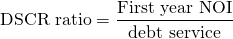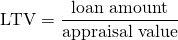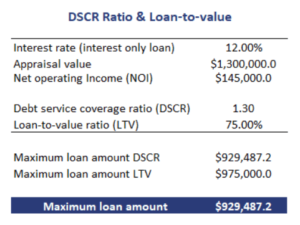# DSCR Ratio

The DSCR ratio or debt service coverage ratio is a measure widely used by banks to determine the maximum loan amount. This maximum loan amount is the maximum amount a bank is willing to lend for a real estate investment. Typically, the maximum loan amount is the lower of the loan-to-value and the level of debt based on the debt service coverage ratio.

On this page, we discuss the DSCR ratio formula, discuss its importance in the real estate sector and finally show how to calculate the DSCR ratio in Excel. The Excel sheet containing the SDCR calculator is available for download at the bottom of the page.

## DSCR ratio formula

The DSCR calculation formula is the followingDebt service includes both the interest and the principal payment and NOI is the net operating income. The calculation of the NOI is discussed in the page on the income approach.

The loan-to-value (LTV) is calculated as follows## Ideal DSCR ratio in real estate

It is hard to say what the ideal DSCR should be. That depends on the riskiness of the enterprise. If the building is both the used by a company that will have predictable, stable revenues, the bank may allow a higher DSCR. If instead, the company is active in a sector with high default rates, the DSCR will likely be lower.

## DSCR ratio example

Next, let’s apply the DSCR calculation formula to a realistic example. Let’s assume we have a piece of property that is worth \$1,300,000.0. In addition, the bank requires a minimum DSCR of 1.3. In that case, what is the maximum amount of money we can borrow to purchase the property? The following table illustrates the necessary calculations.The DSCR calculation in Excel is available below.

## Summary

We discussed how to calculate DSCR in Excel. Very often however, the bank will require a minimum DSCR. In that case, the investors should be able to determine the maximum loan amount. This is the ideal DSCR for a bank loan, as it is the ratio that the banks considers appropriate.

### Calculation in Excel

Want to have an implementation in Excel? Download the Excel file: DSCR Ratio